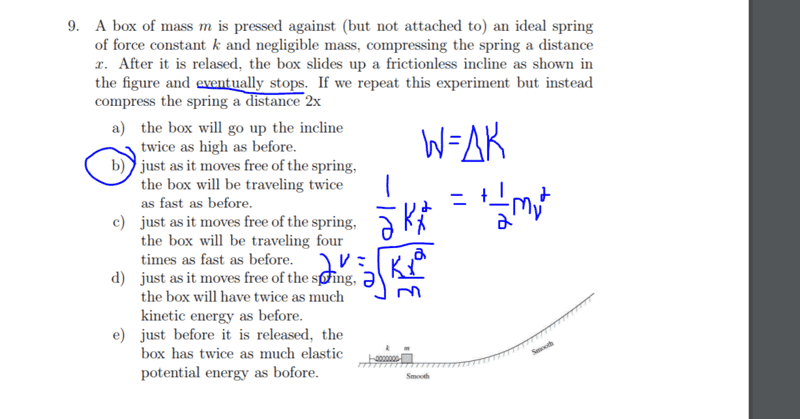# Did i do this problem right? (Work by Spring and KE)

Lori

## Homework StatementW= KE
Wspring = .5kx^2

## The Attempt at a Solution

since W = .5mv^2 = Wspring = .5x^2
i solve for v and get that v = sqrt(kx^2/m)
if i plug in multiply the x by 2, the 2 squares and i get 4. the square root of 4 is just 2 and it comes out as a constant , and so velocity is 2 times faster

Did i go through the problem correctly?[/B]

PeroK
Homework Helper
Gold Member
2020 Award

## Homework Statement

View attachment 214618

W= KE
Wspring = .5kx^2

## The Attempt at a Solution

since W = .5mv^2 = Wspring = .5x^2
i solve for v and get that v = sqrt(kx^2/m)
if i plug in multiply the x by 2, the 2 squares and i get 4. the square root of 4 is just 2 and it comes out as a constant , and so velocity is 2 times faster

Did i go through the problem correctly?[/B]

You got the right answer for the right reasons. You might like to think, however, about how you could organise your working better.

Abhishek kumar

## Homework Statement

View attachment 214618

W= KE
Wspring = .5kx^2

## The Attempt at a Solution

since W = .5mv^2 = Wspring = .5x^2
i solve for v and get that v = sqrt(kx^2/m)
if i plug in multiply the x by 2, the 2 squares and i get 4. the square root of 4 is just 2 and it comes out as a constant , and so velocity is 2 times faster

Did i go through the problem correctly?[/B]
You are right but if you have to write what is relation between heights

Lori
You are right but if you have to write what is relation between heights
mgh + 1/2mv^2=PE of Spring + mgh
mgh + 1/2mv^2 = .5kx^2 + 0
mgh + .5mv^2 = .5k(2x)^2
mgh + .5mv^2 =4 (.5kx^2)

since m and g are constant in the left side of the equation, the coefficient 4 will affect the height and the speed of the object and the final KE
height will be 4 times as big and the velocity would be twice as fast at final, while the final KE will times 4

Abhishek kumar
mgh + 1/2mv^2=PE of Spring + mgh
mgh + 1/2mv^2 = .5kx^2 + 0
mgh + .5mv^2 = .5k(2x)^2
mgh + .5mv^2 =4 (.5kx^2)
height will be 4 times as big and the velocity would be twice as fast at final
When block will reach its maximum height velocity will be zero at that instant simply applying energy conservation at that instant you will get the result

Lori
When block will reach its maximum height velocity will be zero at that instant simply applying energy conservation at that instant you will get the result
How would you write the equation?

How is the one I wrote above incorrect?

In energy conservation E2 = E1

(Final energy) PE2 + KE2 = PE + KE1 (initial energy)

Abhishek kumar
How would you write the equation?

How is the one I wrote above incorrect?

In energy conservation E2 = E1

(Final energy) PE2 + KE2 = PE + KE1 (initial energy)
When block reach max height at that instant velocity seized and no kinetic energy
. 5kx^2=mgh

•Lori
haruspex
Homework Helper
Gold Member
2020 Award
seized
Ceased.

Abhishek kumar
Ceased.
Thank you for correcting me

Lori
Thank you for correcting me
Oh I see. So in this spring problem. The initial energy comes from PE of spring.

The final energy comes from the gravitational energy since kinetic energy is 0 (because the object stops)

•Abhishek kumar
Abhishek kumar
Oh I see. So in this spring problem. The initial energy comes from PE of spring.

The final energy comes from the gravitational energy since kinetic energy is 0 (because the object stops)[
Oh I see. So in this spring problem. The initial energy comes from PE of spring.

The final energy comes from the gravitational energy since kinetic energy is 0 (because the object stops)
Yes you got it